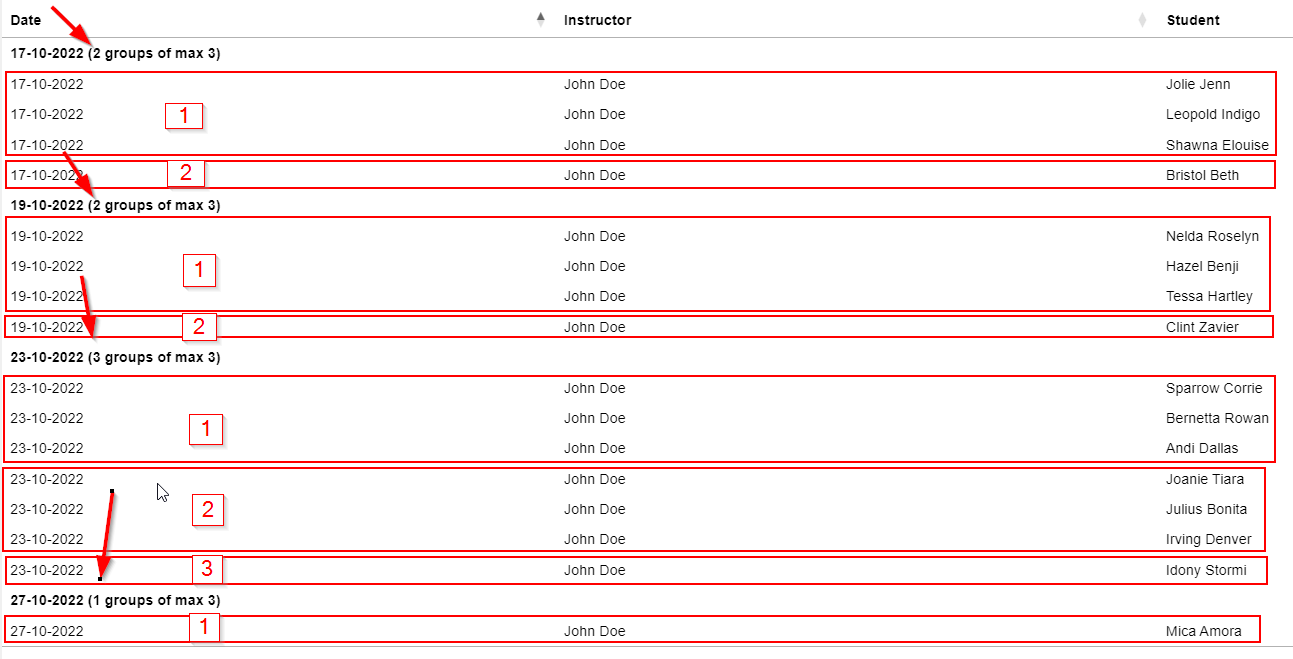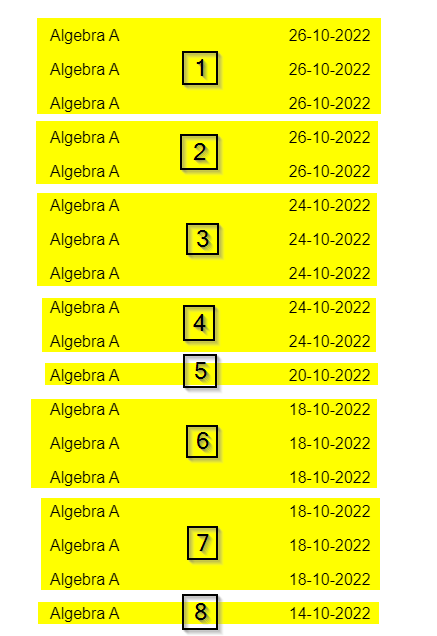# Count in rowGroups

I am trying to customize how the count is working. At the moment i can get count in `rowGroup` to work just fine. You can see an example here.
What i am trying to archive is a count by group of size `3`.

Example
I want to count rows in groups of max 3. So if you look at my example i want to get a count of 2 on the date `17-10-2022`, because we have 4 rows here. We have one group of 3 and one group of 1.

On the date `23-10-2022` i want a count of 3, because we have 2 full group of 3 and 1 group of 1.

In PHP i would `array_chunk` the array i am trying to count, and then return the count of this.
I have created this screenshot to help understand.Hope someone can point me in the right direction• I don't have a Codepen account to save an updated test case but you probably want something like this:

``````            startRender: function (rows, group) {
var numGroups = Math.round( rows.count() / 3 ) + 1;
return group + ' (' + numGroups + ' groups max of 3)';
}
``````

Kevin

• You might want to use `Math.ceil()` instead and remove the `+ 1`. `Math.round()` is probably not the right function to useKevin

• Thanks, that seems to work, but what if i have 2x rowGrouping and wants the count of max 3 per group per date?
I have updated the example.
It should count like this.• When using multiple levels the `rowGroup.startRender` has an additional parameter called `level`. Not sure if that is relevant for your question but you may need to check if its level 0 or 1.

You can use `rows().every()` to loop through all the rows in the group. Are you just counting the number of times the date changes?

Do something like this to iterate the displayed rows in the group:

``````rows.every( function ( rowIdx, tableLoop, rowLoop ) {
var data = this.data();
// ... do something with data(), or this.node(), etc
} );
``````

Kevin

• Are you just counting the number of times the date changes?

I am trying to count the number of classes each instructor has. One class can be max 3 students. When the date changes, the group reset and start a new class.

• If there are 5 studens on the same date, this should count as 2.
• If there is only 1 student on one date, this should count as 1.
• If there are 10 students on the same date, this should count as 4.

You can see the same logic illustrated in the above screenshot.

• Maybe use an object with the key being the date and the value being the number of times the date occurs within the group. Then calculate the number of groups from that. Maybe this pseudo code will give an idea:

``````var studentsPerDate = {};
rows.every( function ( rowIdx, tableLoop, rowLoop ) {
var data = this.data();
// ... do something with data(), or this.node(), etc
if ( ! object.hasOwnProperty( data ) {
studentsPerDate[ data ] = 0;
}
studentsPerDate[ data ]++;  // data is the Date column
} );

// Loop through studentsPerDate to calculate the number of groups.
``````

Kevin